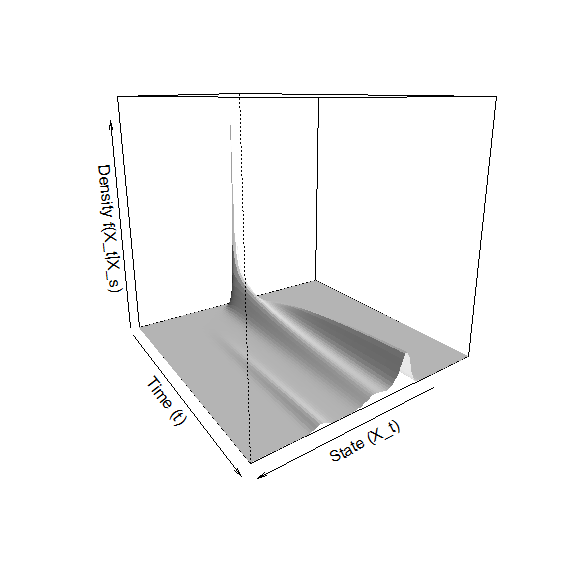# Introduction

Diffusion processes are defined in terms of stochastic differential equations (SDEs), where the dynamics of the process $$X_t$$ is governed by the equation $dX_t = \mu(X_t,t)dt +\sigma(X_t,t)dB_t,$ where $$\mu(X_t,t)$$ denotes the drift coefficient of the process, $$\sigma(X_t,t)$$ denotes the diffusion coefficient of the process and $$B_t$$ is standard Brownian motion. By writing the SDE in integrated form, one can see that the trajectory of the process manifests as the result of a combination of stochastic integrals: $X_t = X_s +\int_s^t \mu(X_u,u)du+\int_s^t \sigma(X_u,u)dB_u.$ Depending on the specification of the drift and diffusion coefficients, the process may exhibit a wide range of interesting dynamical behaviour, making diffusion processes a flexible tool for modelling real-world phenomena. Unfortunately, diffusion processes are notoriously difficult to work with since closed form solutions to SDEs do not exist in general, and calculating probabilistic quantities associated with the process are more often than not analytically intractable. The purpose of the DiffusionRimp is to provide a set of routines in the R environment which can be used to analyse diffusion processes with quite general forms of drift and diffusion coefficients. The package collates a number of routines for performing inference on discretely observed processes using a data-imputation scheme as well as calculating transition densities and first passage time densities using a direct numerical technique known as the method of lines (Hamdi, Schiesser, and Griffiths 2007).

# Interface

The interface of the DiffusionRimp relies on the user to define a diffusion model of interest by assigning functional forms to the coefficients of the SDE. This is achieved by defining functions with predefined names in the workspace in terms of lexical elements that are common in the nomenclature of diffusion processes. For example, the diffusion model:

$dX_t = -\alpha X_t\sin(X_t\pi) dt +\sigma dB_t$

can be defined R-syntax with respect to the template equation $dX_t = \mu(X_t,t)dt +\sigma(X_t,t)dB_t,$ as:

mu  <- function(X,t){-alpha*X*sin(X*pi)}
sig <- function(X,t){sigma}

where $$\mu(X_t,t)$$ = mu(X,t) and $$\sigma(X_t,t)$$ = sig(X,t). From this analysis can be conducted by calling a desired routine. For example, setting $$\alpha = 1$$ and $$\sigma = 0.5$$, we can calculate the transitional density using the MOL.density() function:

library(DiffusionRimp)

alpha <- 1
sigma <- 0.5
res   <- MOL.density(0.1, 0, 5, c(-4, 4), 101, 0.01)

for which we can draw an interesting perspective plot:

persp(x = res$Xt, y = res$time, z = res\$density, col = 'white', xlab = 'State (X_t)',ylab='Time (t)',
zlab='Density f(X_t|X_s)', border = NA, shade = 0.5, theta = 145)Using the various routines of the DiffusionRimp package, we can analyse similarly complex and interesting non-linear diffusion models. These routines are discussed in more detail in the accompanying vignettes and suplementary materials. See the Further reading’’ section for more details.

browseVignettes('DiffusionRimp')